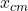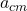Question

Consider the previous situation. Under what condition would the acceleration of the center of mass be zero? Keep in mind that F1x and F2x represent the components, of the corresponding forces. Consider the previous situation. Under what condition would the acceleration of the center of mass be zero? Keep in mind that and represent the components, of the corresponding forces. F1x=−F2x F1x=F2x m1=m2 m1≪m2

1.trucchi

a) m₁ = m₂  F₁ₓ = F₂ₓ

b) m₁ << m₂   F₂ₓ =0

Explanation:

This interesting exercise is unclear your statement, so that in a center of mass system has an acceleration of zero it is necessary that the sum of the forces on each axis is zero, to see this we write Newton’s second law

∑ F = m a

for acceleration to be zero implies that the net force is zero.

we must write the expression for the center of mass= 1 / M (m₁ x₁ + m₂ x₂)

now let’s use the derivatives= d² x_{cm}/dt² = 1 / M (m₁ a₁ + m₂a₂)

where M is the total mass M = m₁ + m₂

so that the acceleration of the center of mass is zero

0 = 1 / M (m₁ a₁ + m₂a₂)

m₁ a₁ = – m₂ a₂

In the case that we have components on the x axis, the modulus of the two forces are equal and their direction is opposite, therefore

F₁ₓ = -F₂ₓ

b)r when the two masses are equal
, in the case of a mass greater than the other m₁ << m₂

acm = d2 xcm / dt2 = 1 / M (m1 a1 + m2a2)

so that the acceleration of the center of mass is zero

0 = 1 / M (m1 a1 + m2a2)

m1 a1 = – m 2 a2

with the initial condition, we can despise m₁, therefore

0 = m₂a₂

if we use Newton’s second law

F₂ = 0

I tell you that in this case with a very high mass difference the force on the largest mass must be almost zero